# 吴恩达深度学习课程deeplearning.ai课程作业：Class 4 Week 3 Car detection

1. 评论中总有人问为什么直接复制这些notebook运行不了？请不要直接复制粘贴，不可能运行通过的，这个只是notebook中我们要自己写的那部分，要正确运行还需要其他py文件，请自己到GitHub上下载完整的。这里的部分仅仅是参考用的，建议还是自己按照提示一点一点写，如果实在卡住了再看答案。个人觉得这样才是正确的学习方法，况且作业也不算难。
2. 关于评论中有人说我是抄袭，注释还没别人详细，复制下来还运行不过。答复是：做伸手党之前，请先搞清这个作业是干什么的。大家都是从GitHub上下载原始的作业，然后根据代码前面的提示（通常会指定函数和公式）来编写代码，而且后面还有expected output供你比对，如果程序正确，结果一般来说是一样的。请不要无脑喷，说什么跟别人的答案一样的。说到底，我们要做的就是，看他的文字部分，根据提示在代码中加入部分自己的代码。我们自己要写的部分只有那么一小部分代码。
3. 由于实在很反感无脑喷子，故禁止了下面的评论功能，请见谅。如果有问题，请私信我，在力所能及的范围内会尽量帮忙。

# 准备工作：

### 配置环境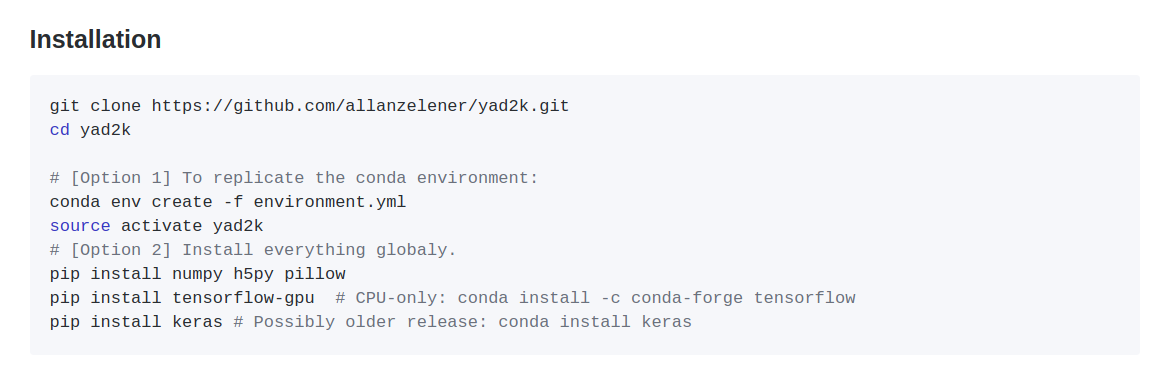### 下载已经训练好的模型的cfg和weights文件：

wget http://pjreddie.com/media/files/yolo.weights
wget https://raw.githubusercontent.com/pjreddie/darknet/master/cfg/yolo.cfg

### 生成我们想要的h5文件

python3 yad2k.py yolo.cfg yolo.weights model_data/yolo.h5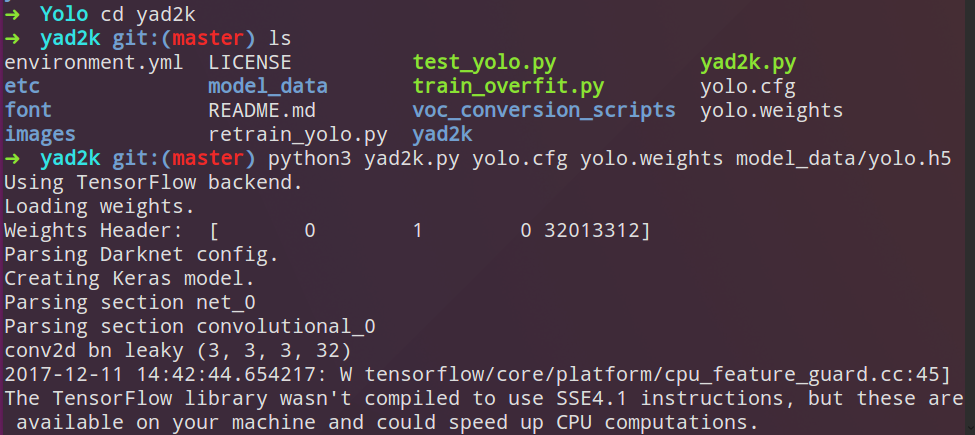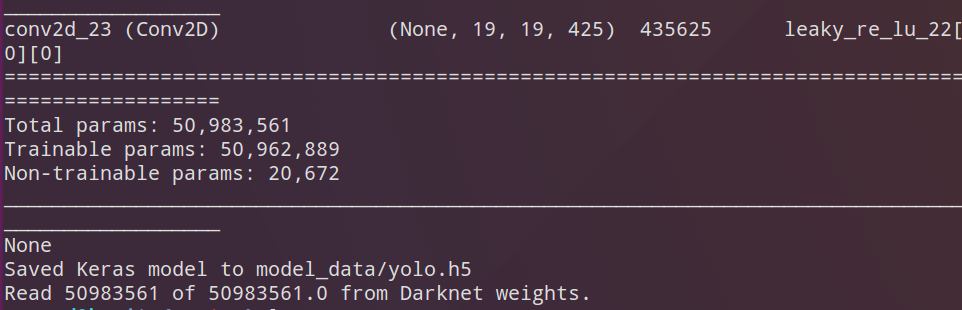# Autonomous driving - Car detection

Welcome to your week 3 programming assignment. You will learn about object detection using the very powerful YOLO model. Many of the ideas in this notebook are described in the two YOLO papers: Redmon et al., 2016 (https://arxiv.org/abs/1506.02640) and Redmon and Farhadi, 2016 (https://arxiv.org/abs/1612.08242).

You will learn to:
- Use object detection on a car detection dataset
- Deal with bounding boxes

Run the following cell to load the packages and dependencies that are going to be useful for your journey!

import argparse
import os
import matplotlib.pyplot as plt
from matplotlib.pyplot import imshow
import scipy.io
import scipy.misc
import numpy as np
import pandas as pd
import PIL
import tensorflow as tf
from keras import backend as K
from keras.layers import Input, Lambda, Conv2D
from keras.models import load_model, Model
from yolo_utils import read_classes, read_anchors, generate_colors, preprocess_image, draw_boxes, scale_boxes
from yad2k.models.keras_yolo import yolo_head, yolo_boxes_to_corners, preprocess_true_boxes, yolo_loss, yolo_body

%matplotlib inline
Using TensorFlow backend.


Important Note: As you can see, we import Keras’s backend as K. This means that to use a Keras function in this notebook, you will need to write: K.function(...).

## 1 - Problem Statement

You are working on a self-driving car. As a critical component of this project, you’d like to first build a car detection system. To collect data, you’ve mounted a camera to the hood (meaning the front) of the car, which takes pictures of the road ahead every few seconds while you drive around.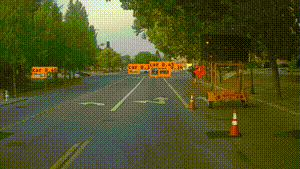Pictures taken from a car-mounted camera while driving around Silicon Valley.
We would like to especially thank drive.ai for providing this dataset! Drive.ai is a company building the brains of self-driving vehicles.You’ve gathered all these images into a folder and have labelled them by drawing bounding boxes around every car you found. Here’s an example of what your bounding boxes look like.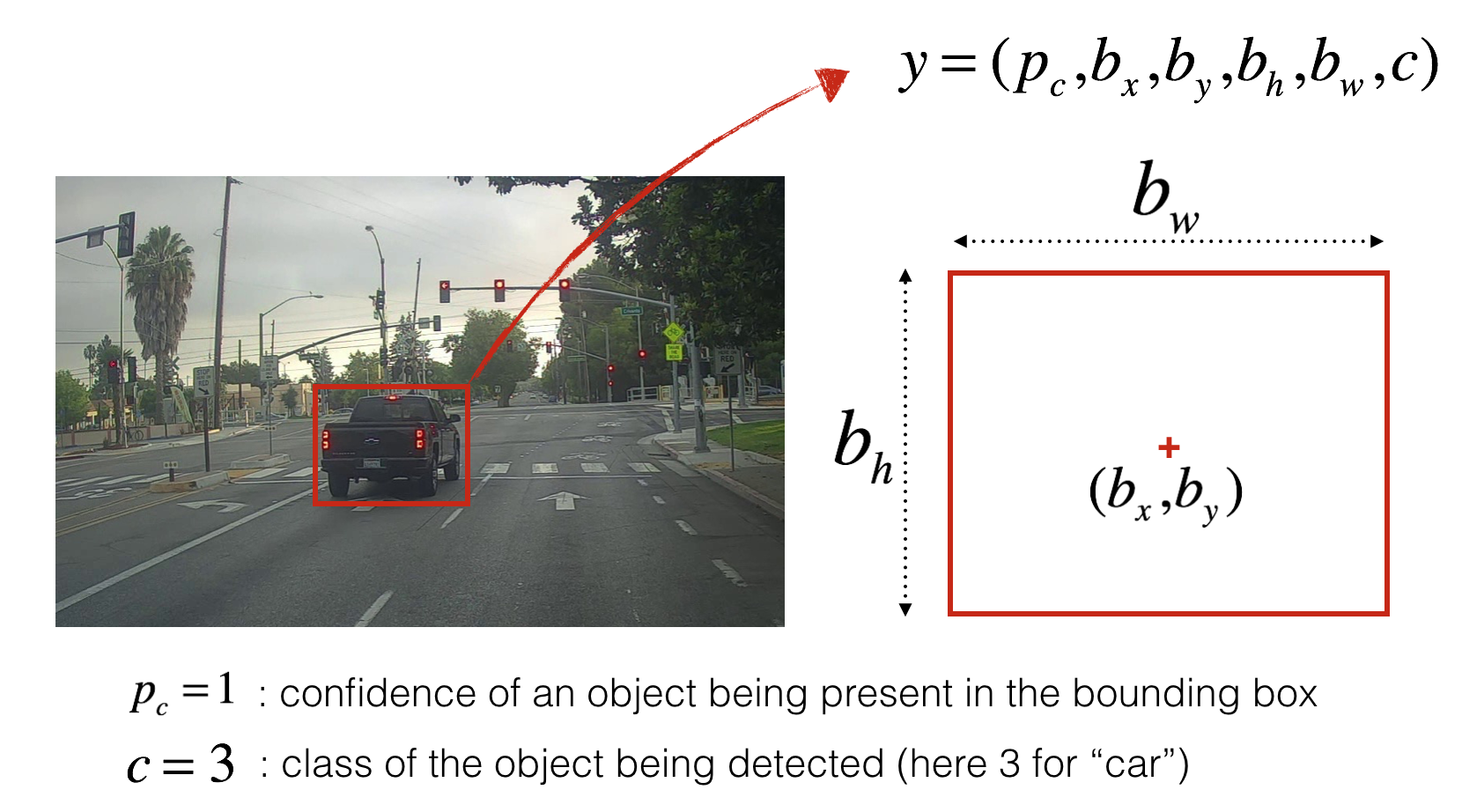Figure 1 : Definition of a box

If you have 80 classes that you want YOLO to recognize, you can represent the class label $c$$c$ either as an integer from 1 to 80, or as an 80-dimensional vector (with 80 numbers) one component of which is 1 and the rest of which are 0. The video lectures had used the latter representation; in this notebook, we will use both representations, depending on which is more convenient for a particular step.

In this exercise, you will learn how YOLO works, then apply it to car detection. Because the YOLO model is very computationally expensive to train, we will load pre-trained weights for you to use.

## 2 - YOLO

YOLO (“you only look once”) is a popular algoritm because it achieves high accuracy while also being able to run in real-time. This algorithm “only looks once” at the image in the sense that it requires only one forward propagation pass through the network to make predictions. After non-max suppression, it then outputs recognized objects together with the bounding boxes.

### 2.1 - Model details

First things to know:
- The input is a batch of images of shape (m, 608, 608, 3)
- The output is a list of bounding boxes along with the recognized classes. Each bounding box is represented by 6 numbers $\left({p}_{c},{b}_{x},{b}_{y},{b}_{h},{b}_{w},c\right)$$(p_c, b_x, b_y, b_h, b_w, c)$ as explained above. If you expand $c$$c$ into an 80-dimensional vector, each bounding box is then represented by 85 numbers.

We will use 5 anchor boxes. So you can think of the YOLO architecture as the following: IMAGE (m, 608, 608, 3) -> DEEP CNN -> ENCODING (m, 19, 19, 5, 85).

Lets look in greater detail at what this encoding represents.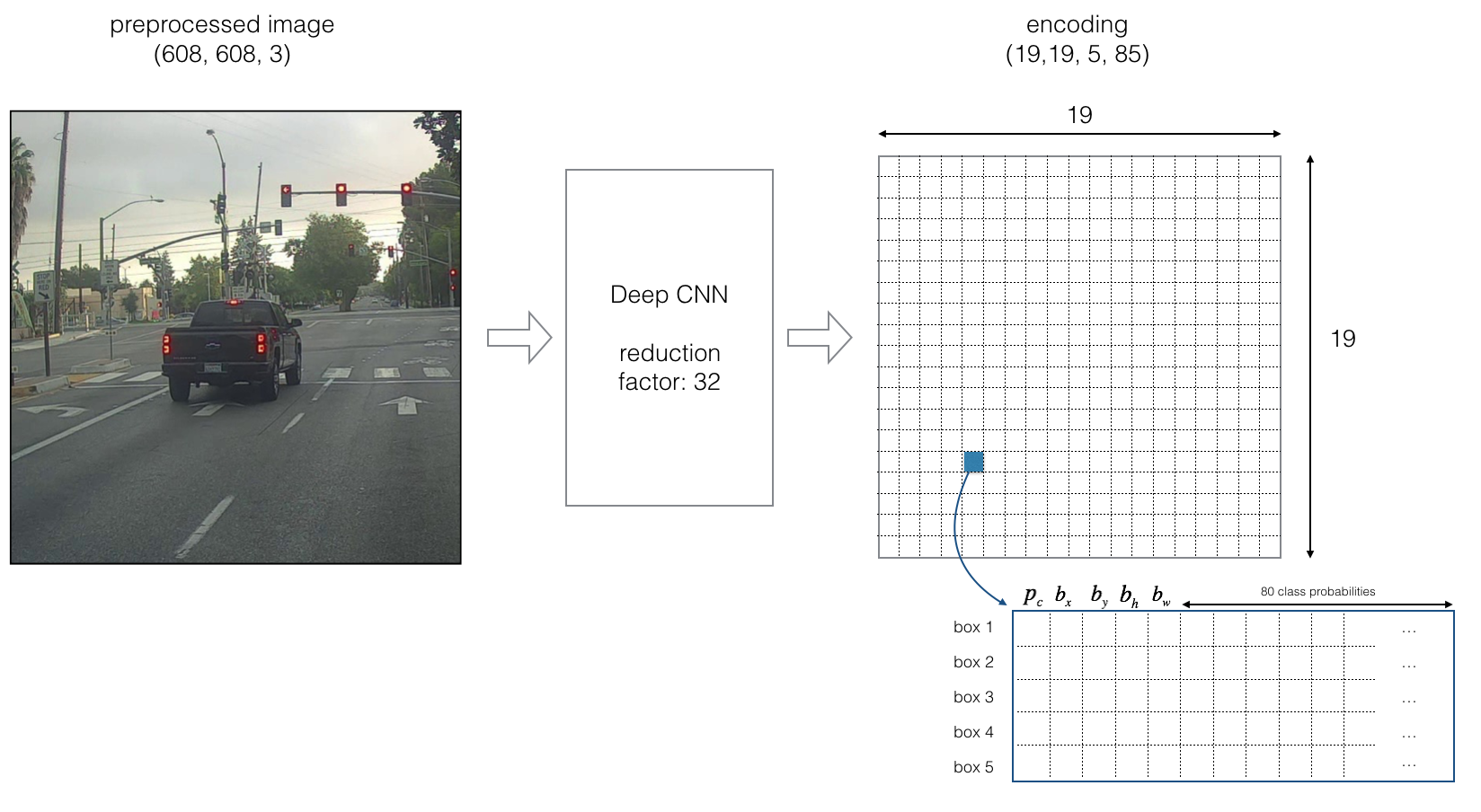Figure 2 : Encoding architecture for YOLO

If the center/midpoint of an object falls into a grid cell, that grid cell is responsible for detecting that object.

Since we are using 5 anchor boxes, each of the 19 x19 cells thus encodes information about 5 boxes. Anchor boxes are defined only by their width and height.

For simplicity, we will flatten the last two last dimensions of the shape (19, 19, 5, 85) encoding. So the output of the Deep CNN is (19, 19, 425).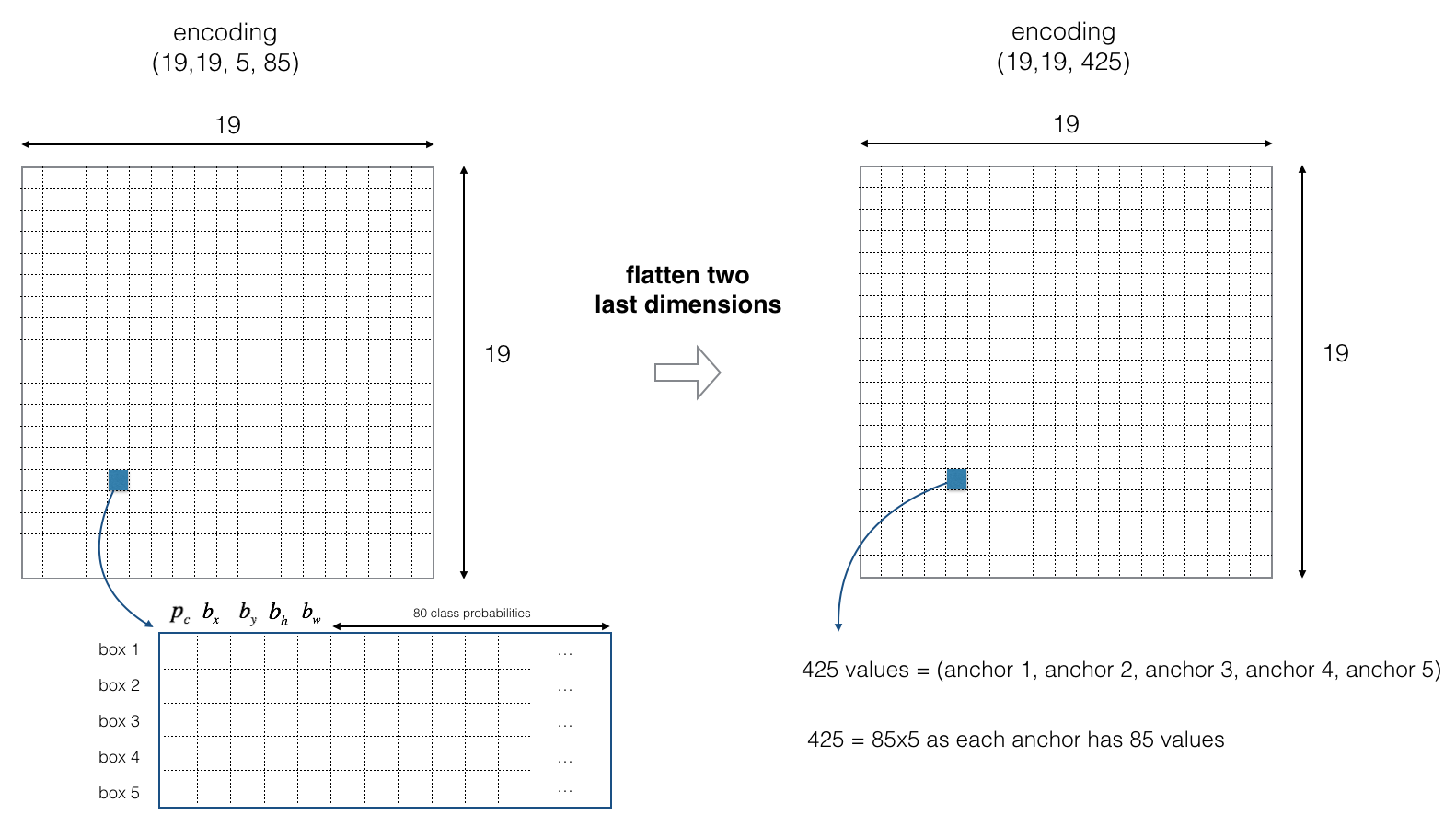Figure 3 : Flattening the last two last dimensions

Now, for each box (of each cell) we will compute the following elementwise product and extract a probability that the box contains a certain class.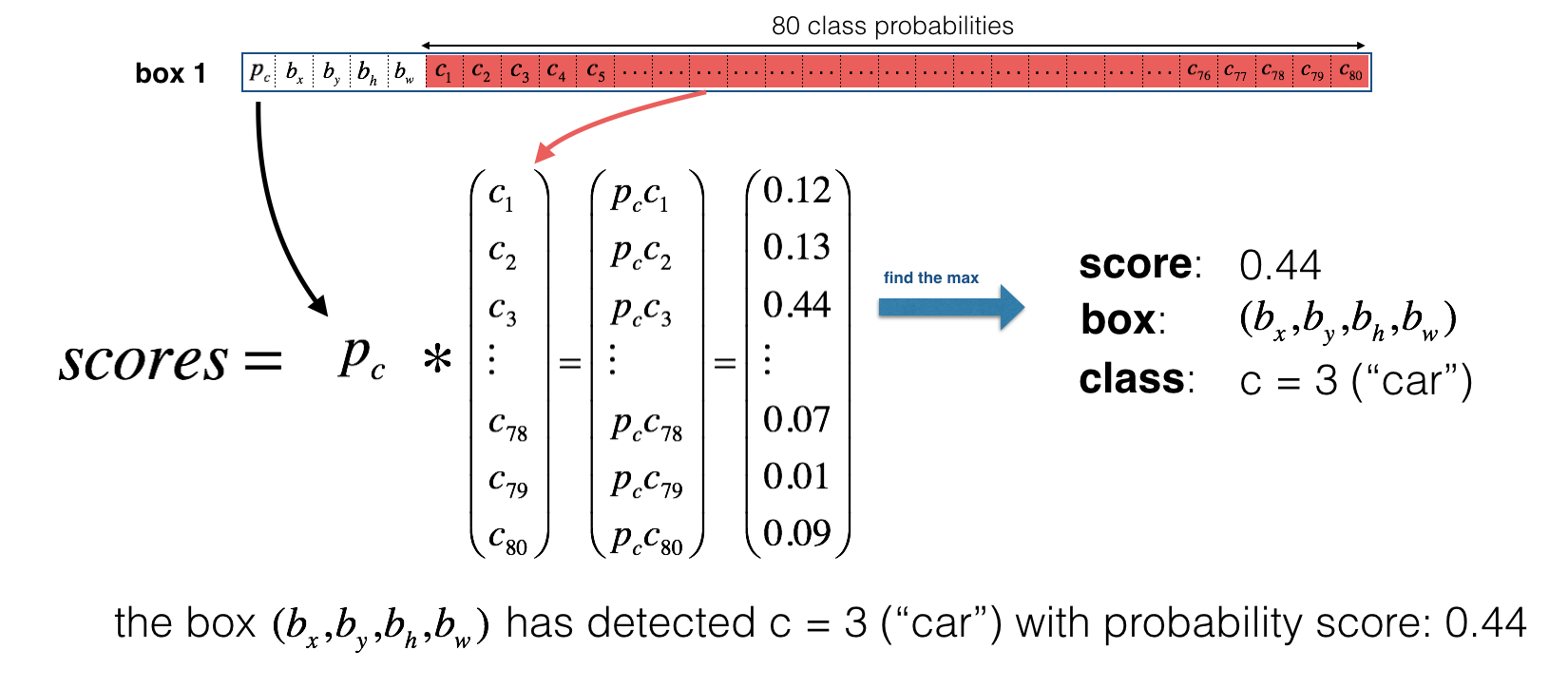Figure 4 : Find the class detected by each box

Here’s one way to visualize what YOLO is predicting on an image:
- For each of the 19x19 grid cells, find the maximum of the probability scores (taking a max across both the 5 anchor boxes and across different classes).
- Color that grid cell according to what object that grid cell considers the most likely.

Doing this results in this picture: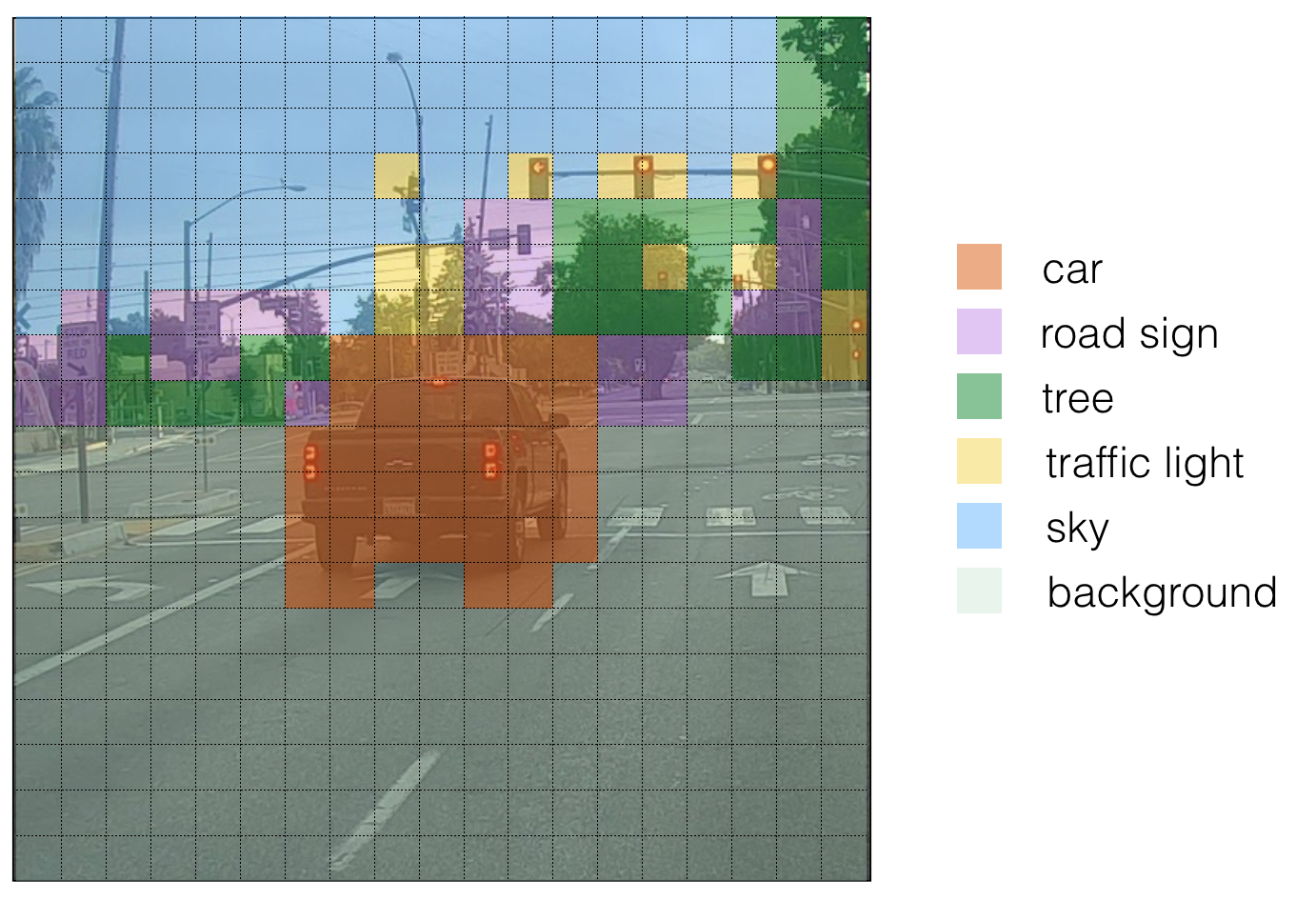Figure 5 : Each of the 19x19 grid cells colored according to which class has the largest predicted probability in that cell.

Note that this visualization isn’t a core part of the YOLO algorithm itself for making predictions; it’s just a nice way of visualizing an intermediate result of the algorithm.

Another way to visualize YOLO’s output is to plot the bounding boxes that it outputs. Doing that results in a visualization like this: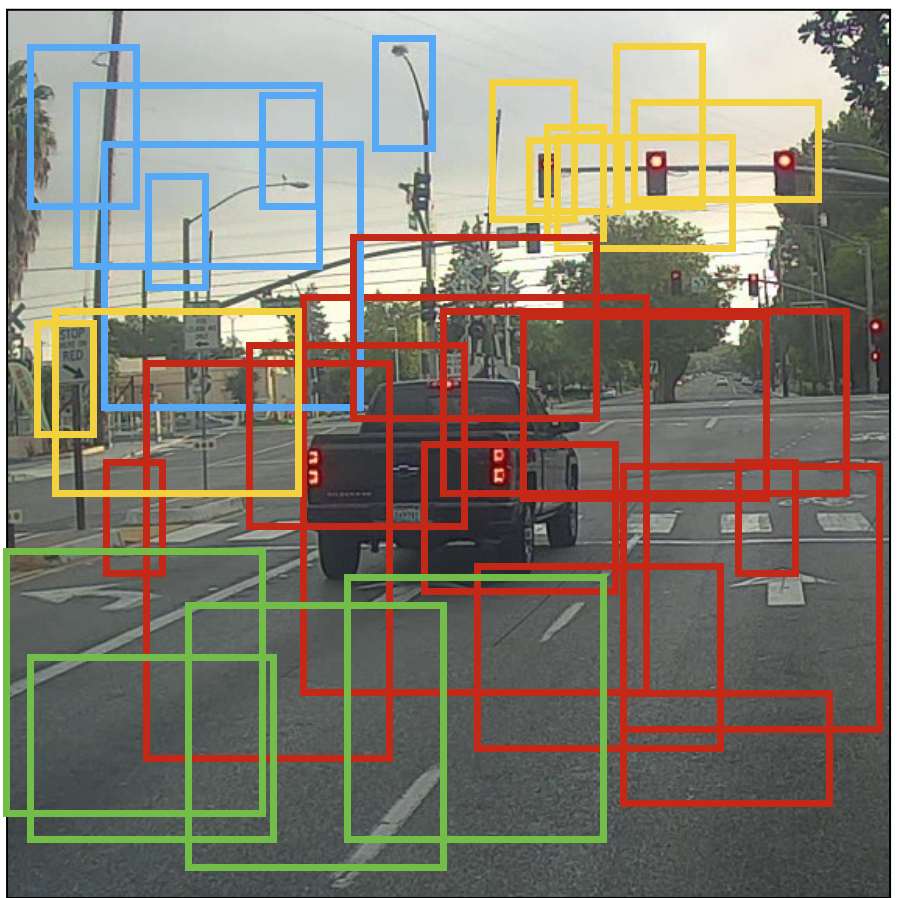Figure 6 : Each cell gives you 5 boxes. In total, the model predicts: 19x19x5 = 1805 boxes just by looking once at the image (one forward pass through the network)! Different colors denote different classes.

In the figure above, we plotted only boxes that the model had assigned a high probability to, but this is still too many boxes. You’d like to filter the algorithm’s output down to a much smaller number of detected objects. To do so, you’ll use non-max suppression. Specifically, you’ll carry out these steps:
- Get rid of boxes with a low score (meaning, the box is not very confident about detecting a class)
- Select only one box when several boxes overlap with each other and detect the same object.

### 2.2 - Filtering with a threshold on class scores

You are going to apply a first filter by thresholding. You would like to get rid of any box for which the class “score” is less than a chosen threshold.

The model gives you a total of 19x19x5x85 numbers, with each box described by 85 numbers. It’ll be convenient to rearrange the (19,19,5,85) (or (19,19,425)) dimensional tensor into the following variables:
- box_confidence: tensor of shape $\left(19×19,5,1\right)$$(19 \times 19, 5, 1)$ containing ${p}_{c}$$p_c$ (confidence probability that there’s some object) for each of the 5 boxes predicted in each of the 19x19 cells.
- boxes: tensor of shape $\left(19×19,5,4\right)$$(19 \times 19, 5, 4)$ containing $\left({b}_{x},{b}_{y},{b}_{h},{b}_{w}\right)$$(b_x, b_y, b_h, b_w)$ for each of the 5 boxes per cell.
- box_class_probs: tensor of shape $\left(19×19,5,80\right)$$(19 \times 19, 5, 80)$ containing the detection probabilities $\left({c}_{1},{c}_{2},...{c}_{80}\right)$$(c_1, c_2, ... c_{80})$ for each of the 80 classes for each of the 5 boxes per cell.

Exercise: Implement yolo_filter_boxes().
1. Compute box scores by doing the elementwise product as described in Figure 4. The following code may help you choose the right operator:

a = np.random.randn(19*19, 5, 1)
b = np.random.randn(19*19, 5, 80)
c = a * b # shape of c will be (19*19, 5, 80)
1. For each box, find:
• the index of the class with the maximum box score (Hint) (Be careful with what axis you choose; consider using axis=-1)
• the corresponding box score (Hint) (Be careful with what axis you choose; consider using axis=-1)
2. Create a mask by using a threshold. As a reminder: ([0.9, 0.3, 0.4, 0.5, 0.1] < 0.4) returns: [False, True, False, False, True]. The mask should be True for the boxes you want to keep.
3. Use TensorFlow to apply the mask to box_class_scores, boxes and box_classes to filter out the boxes we don’t want. You should be left with just the subset of boxes you want to keep. (Hint)

Reminder: to call a Keras function, you should use K.function(...).

# GRADED FUNCTION: yolo_filter_boxes

def yolo_filter_boxes(box_confidence, boxes, box_class_probs, threshold = .6):
"""Filters YOLO boxes by thresholding on object and class confidence.

Arguments:
box_confidence -- tensor of shape (19, 19, 5, 1)
boxes -- tensor of shape (19, 19, 5, 4)
box_class_probs -- tensor of shape (19, 19, 5, 80)
threshold -- real value, if [ highest class probability score < threshold], then get rid of the corresponding box

Returns:
scores -- tensor of shape (None,), containing the class probability score for selected boxes
boxes -- tensor of shape (None, 4), containing (b_x, b_y, b_h, b_w) coordinates of selected boxes
classes -- tensor of shape (None,), containing the index of the class detected by the selected boxes

Note: "None" is here because you don't know the exact number of selected boxes, as it depends on the threshold.
For example, the actual output size of scores would be (10,) if there are 10 boxes.
"""

# Step 1: Compute box scores
### START CODE HERE ### (≈ 1 line)
box_scores = box_confidence * box_class_probs
### END CODE HERE ###

# Step 2: Find the box_classes thanks to the max box_scores, keep track of the corresponding score
### START CODE HERE ### (≈ 2 lines)
box_classes = K.argmax(box_scores, axis=-1)
box_class_scores = K.max(box_scores, axis=-1, keepdims=False)
#     print(box_classes.shape)
#     print(box_class_scores.shape)
### END CODE HERE ###

# Step 3: Create a filtering mask based on "box_class_scores" by using "threshold". The mask should have the
# same dimension as box_class_scores, and be True for the boxes you want to keep (with probability >= threshold)
### START CODE HERE ### (≈ 1 line)
filtering_mask = box_class_scores >= threshold
### END CODE HERE ###

# Step 4: Apply the mask to scores, boxes and classes
### START CODE HERE ### (≈ 3 lines)
#     print(box_class_scores.shape)
#     print(filtering_mask.shape)
scores = tf.boolean_mask(box_class_scores, filtering_mask)
boxes = tf.boolean_mask(boxes, filtering_mask)
classes = tf.boolean_mask(box_classes, filtering_mask)
### END CODE HERE ###

return scores, boxes, classes
with tf.Session() as test_a:
box_confidence = tf.random_normal([19, 19, 5, 1], mean=1, stddev=4, seed = 1)
boxes = tf.random_normal([19, 19, 5, 4], mean=1, stddev=4, seed = 1)
box_class_probs = tf.random_normal([19, 19, 5, 80], mean=1, stddev=4, seed = 1)
scores, boxes, classes = yolo_filter_boxes(box_confidence, boxes, box_class_probs, threshold = 0.5)
print("scores = " + str(scores.eval()))
print("boxes = " + str(boxes.eval()))
print("classes = " + str(classes.eval()))
print("scores.shape = " + str(scores.shape))
print("boxes.shape = " + str(boxes.shape))
print("classes.shape = " + str(classes.shape))
scores = 10.7506
boxes = [ 8.42653275  3.27136683 -0.5313437  -4.94137383]
classes = 7
scores.shape = (?,)
boxes.shape = (?, 4)
classes.shape = (?,)


Expected Output:

 scores 10.7506 boxes [ 8.42653275 3.27136683 -0.5313437 -4.94137383] classes 7 scores.shape (?,) boxes.shape (?, 4) classes.shape (?,)

### 2.3 - Non-max suppression

Even after filtering by thresholding over the classes scores, you still end up a lot of overlapping boxes. A second filter for selecting the right boxes is called non-maximum suppression (NMS).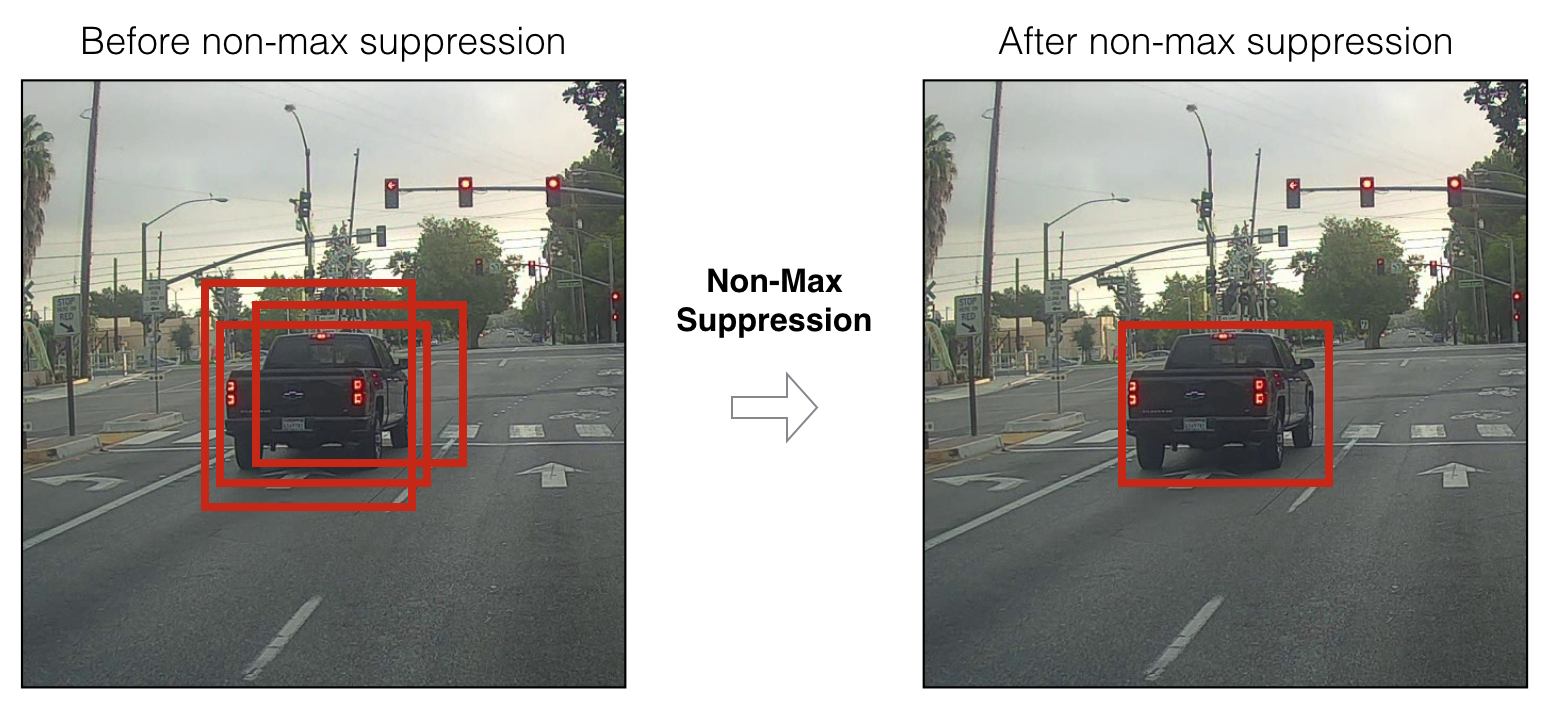Figure 7 : In this example, the model has predicted 3 cars, but it’s actually 3 predictions of the same car. Running non-max suppression (NMS) will select only the most accurate (highest probabiliy) one of the 3 boxes.

Non-max suppression uses the very important function called “Intersection over Union”, or IoU.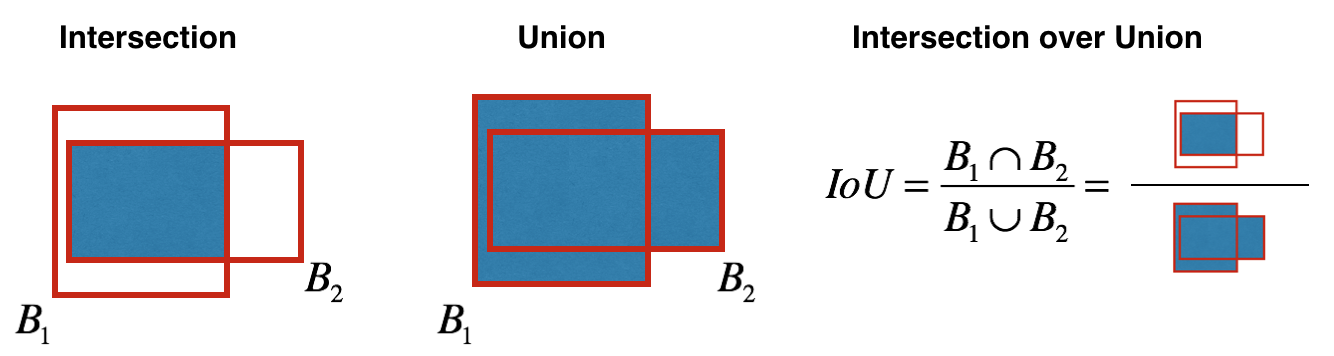Figure 8 : Definition of “Intersection over Union”.

Exercise: Implement iou(). Some hints:
- In this exercise only, we define a box using its two corners (upper left and lower right): (x1, y1, x2, y2) rather than the midpoint and height/width.
- To calculate the area of a rectangle you need to multiply its height (y2 - y1) by its width (x2 - x1)
- You’ll also need to find the coordinates (xi1, yi1, xi2, yi2) of the intersection of two boxes. Remember that:
- xi1 = maximum of the x1 coordinates of the two boxes
- yi1 = maximum of the y1 coordinates of the two boxes
- xi2 = minimum of the x2 coordinates of the two boxes
- yi2 = minimum of the y2 coordinates of the two boxes

In this code, we use the convention that (0,0) is the top-left corner of an image, (1,0) is the upper-right corner, and (1,1) the lower-right corner.

# GRADED FUNCTION: iou

def iou(box1, box2):
"""Implement the intersection over union (IoU) between box1 and box2

Arguments:
box1 -- first box, list object with coordinates (x1, y1, x2, y2)
box2 -- second box, list object with coordinates (x1, y1, x2, y2)
"""

# Calculate the (y1, x1, y2, x2) coordinates of the intersection of box1 and box2. Calculate its Area.
### START CODE HERE ### (≈ 5 lines)
xi1 = max(box1, box2)
yi1 = max(box1, box2)
xi2 = min(box1, box2)
yi2 = min(box1, box2)
inter_area = (xi2 - xi1) * (yi2 - yi1)
#     print(xi1, yi1, xi2, yi2)
#     print(inter_area)
### END CODE HERE ###

# Calculate the Union area by using Formula: Union(A,B) = A + B - Inter(A,B)
### START CODE HERE ### (≈ 3 lines)
box1_area = (box1 - box1) * (box1 - box1)
box2_area = (box2 - box2) * (box2 - box2)
union_area = box1_area + box2_area - inter_area
#     print(union_area)
### END CODE HERE ###

# compute the IoU
### START CODE HERE ### (≈ 1 line)
iou = float(inter_area) / float(union_area)
### END CODE HERE ###

return iou
box1 = (2, 1, 4, 3)
box2 = (1, 2, 3, 4)
print("iou = " + str(iou(box1, box2)))
iou = 0.14285714285714285


Expected Output:

 iou = 0.142857

You are now ready to implement non-max suppression. The key steps are:
1. Select the box that has the highest score.
2. Compute its overlap with all other boxes, and remove boxes that overlap it more than iou_threshold.
3. Go back to step 1 and iterate until there’s no more boxes with a lower score than the current selected box.

This will remove all boxes that have a large overlap with the selected boxes. Only the “best” boxes remain.

Exercise: Implement yolo_non_max_suppression() using TensorFlow. TensorFlow has two built-in functions that are used to implement non-max suppression (so you don’t actually need to use your iou() implementation):
- tf.image.non_max_suppression()
- K.gather()

# GRADED FUNCTION: yolo_non_max_suppression

def yolo_non_max_suppression(scores, boxes, classes, max_boxes = 10, iou_threshold = 0.5):
"""
Applies Non-max suppression (NMS) to set of boxes

Arguments:
scores -- tensor of shape (None,), output of yolo_filter_boxes()
boxes -- tensor of shape (None, 4), output of yolo_filter_boxes() that have been scaled to the image size (see later)
classes -- tensor of shape (None,), output of yolo_filter_boxes()
max_boxes -- integer, maximum number of predicted boxes you'd like
iou_threshold -- real value, "intersection over union" threshold used for NMS filtering

Returns:
scores -- tensor of shape (, None), predicted score for each box
boxes -- tensor of shape (4, None), predicted box coordinates
classes -- tensor of shape (, None), predicted class for each box

Note: The "None" dimension of the output tensors has obviously to be less than max_boxes. Note also that this
function will transpose the shapes of scores, boxes, classes. This is made for convenience.
"""

max_boxes_tensor = K.variable(max_boxes, dtype='int32')     # tensor to be used in tf.image.non_max_suppression()
K.get_session().run(tf.variables_initializer([max_boxes_tensor])) # initialize variable max_boxes_tensor

# Use tf.image.non_max_suppression() to get the list of indices corresponding to boxes you keep
### START CODE HERE ### (≈ 1 line)
nms_indices = tf.image.non_max_suppression(boxes, scores, max_boxes, iou_threshold)
### END CODE HERE ###

# Use K.gather() to select only nms_indices from scores, boxes and classes
### START CODE HERE ### (≈ 3 lines)
scores = K.gather(scores, nms_indices)
boxes = K.gather(boxes, nms_indices)
classes = K.gather(classes, nms_indices)
### END CODE HERE ###

return scores, boxes, classes
with tf.Session() as test_b:
scores = tf.random_normal([54,], mean=1, stddev=4, seed = 1)
boxes = tf.random_normal([54, 4], mean=1, stddev=4, seed = 1)
classes = tf.random_normal([54,], mean=1, stddev=4, seed = 1)
scores, boxes, classes = yolo_non_max_suppression(scores, boxes, classes)
print("scores = " + str(scores.eval()))
print("boxes = " + str(boxes.eval()))
print("classes = " + str(classes.eval()))
print("scores.shape = " + str(scores.eval().shape))
print("boxes.shape = " + str(boxes.eval().shape))
print("classes.shape = " + str(classes.eval().shape))
scores = 6.9384
boxes = [-5.299932    3.13798141  4.45036697  0.95942086]
classes = -2.24527
scores.shape = (10,)
boxes.shape = (10, 4)
classes.shape = (10,)


Expected Output:

 scores 6.9384 boxes [-5.299932 3.13798141 4.45036697 0.95942086] classes -2.24527 scores.shape (10,) boxes.shape (10, 4) classes.shape (10,)

### 2.4 Wrapping up the filtering

It’s time to implement a function taking the output of the deep CNN (the 19x19x5x85 dimensional encoding) and filtering through all the boxes using the functions you’ve just implemented.

Exercise: Implement yolo_eval() which takes the output of the YOLO encoding and filters the boxes using score threshold and NMS. There’s just one last implementational detail you have to know. There’re a few ways of representing boxes, such as via their corners or via their midpoint and height/width. YOLO converts between a few such formats at different times, using the following functions (which we have provided):

boxes = yolo_boxes_to_corners(box_xy, box_wh) 

which converts the yolo box coordinates (x,y,w,h) to box corners’ coordinates (x1, y1, x2, y2) to fit the input of yolo_filter_boxes

boxes = scale_boxes(boxes, image_shape)

YOLO’s network was trained to run on 608x608 images. If you are testing this data on a different size image–for example, the car detection dataset had 720x1280 images–this step rescales the boxes so that they can be plotted on top of the original 720x1280 image.

Don’t worry about these two functions; we’ll show you where they need to be called.

# GRADED FUNCTION: yolo_eval

def yolo_eval(yolo_outputs, image_shape = (720., 1280.), max_boxes=10, score_threshold=.6, iou_threshold=.5):
"""
Converts the output of YOLO encoding (a lot of boxes) to your predicted boxes along with their scores, box coordinates and classes.

Arguments:
yolo_outputs -- output of the encoding model (for image_shape of (608, 608, 3)), contains 4 tensors:
box_confidence: tensor of shape (None, 19, 19, 5, 1)
box_xy: tensor of shape (None, 19, 19, 5, 2)
box_wh: tensor of shape (None, 19, 19, 5, 2)
box_class_probs: tensor of shape (None, 19, 19, 5, 80)
image_shape -- tensor of shape (2,) containing the input shape, in this notebook we use (608., 608.) (has to be float32 dtype)
max_boxes -- integer, maximum number of predicted boxes you'd like
score_threshold -- real value, if [ highest class probability score < threshold], then get rid of the corresponding box
iou_threshold -- real value, "intersection over union" threshold used for NMS filtering

Returns:
scores -- tensor of shape (None, ), predicted score for each box
boxes -- tensor of shape (None, 4), predicted box coordinates
classes -- tensor of shape (None,), predicted class for each box
"""

### START CODE HERE ###

# Retrieve outputs of the YOLO model (≈1 line)
box_confidence, box_xy, box_wh, box_class_probs = yolo_outputs

# Convert boxes to be ready for filtering functions
boxes = yolo_boxes_to_corners(box_xy, box_wh)

# Use one of the functions you've implemented to perform Score-filtering with a threshold of score_threshold (≈1 line)
scores, boxes, classes = yolo_filter_boxes(box_confidence, boxes, box_class_probs, threshold = .6)

# Scale boxes back to original image shape.
boxes = scale_boxes(boxes, image_shape)

# Use one of the functions you've implemented to perform Non-max suppression with a threshold of iou_threshold (≈1 line)
scores, boxes, classes = yolo_non_max_suppression(scores, boxes, classes, max_boxes = 10, iou_threshold = 0.5)

### END CODE HERE ###

return scores, boxes, classes
with tf.Session() as test_b:
yolo_outputs = (tf.random_normal([19, 19, 5, 1], mean=1, stddev=4, seed = 1),
tf.random_normal([19, 19, 5, 2], mean=1, stddev=4, seed = 1),
tf.random_normal([19, 19, 5, 2], mean=1, stddev=4, seed = 1),
tf.random_normal([19, 19, 5, 80], mean=1, stddev=4, seed = 1))
scores, boxes, classes = yolo_eval(yolo_outputs)
print("scores = " + str(scores.eval()))
print("boxes = " + str(boxes.eval()))
print("classes = " + str(classes.eval()))
print("scores.shape = " + str(scores.eval().shape))
print("boxes.shape = " + str(boxes.eval().shape))
print("classes.shape = " + str(classes.eval().shape))
scores = 138.791
boxes = [ 1292.32971191  -278.52166748  3876.98925781  -835.56494141]
classes = 54
scores.shape = (10,)
boxes.shape = (10, 4)
classes.shape = (10,)


Expected Output:

 scores 138.791 boxes [ 1292.32971191 -278.52166748 3876.98925781 -835.56494141] classes 54 scores.shape (10,) boxes.shape (10, 4) classes.shape (10,)

Summary for YOLO:
- Input image (608, 608, 3)
- The input image goes through a CNN, resulting in a (19,19,5,85) dimensional output.
- After flattening the last two dimensions, the output is a volume of shape (19, 19, 425):
- Each cell in a 19x19 grid over the input image gives 425 numbers.
- 425 = 5 x 85 because each cell contains predictions for 5 boxes, corresponding to 5 anchor boxes, as seen in lecture.
- 85 = 5 + 80 where 5 is because $\left({p}_{c},{b}_{x},{b}_{y},{b}_{h},{b}_{w}\right)$$(p_c, b_x, b_y, b_h, b_w)$ has 5 numbers, and and 80 is the number of classes we’d like to detect
- You then select only few boxes based on:
- Score-thresholding: throw away boxes that have detected a class with a score less than the threshold
- Non-max suppression: Compute the Intersection over Union and avoid selecting overlapping boxes
- This gives you YOLO’s final output.

## 3 - Test YOLO pretrained model on images

In this part, you are going to use a pretrained model and test it on the car detection dataset. As usual, you start by creating a session to start your graph. Run the following cell.

sess = K.get_session()

### 3.1 - Defining classes, anchors and image shape.

Recall that we are trying to detect 80 classes, and are using 5 anchor boxes. We have gathered the information about the 80 classes and 5 boxes in two files “coco_classes.txt” and “yolo_anchors.txt”. Let’s load these quantities into the model by running the next cell.

The car detection dataset has 720x1280 images, which we’ve pre-processed into 608x608 images.

class_names = read_classes("model_data/coco_classes.txt")
anchors = read_anchors("model_data/yolo_anchors.txt")
image_shape = (720., 1280.)    

### 3.2 - Loading a pretrained model

Training a YOLO model takes a very long time and requires a fairly large dataset of labelled bounding boxes for a large range of target classes. You are going to load an existing pretrained Keras YOLO model stored in “yolo.h5”. (These weights come from the official YOLO website, and were converted using a function written by Allan Zelener. References are at the end of this notebook. Technically, these are the parameters from the “YOLOv2” model, but we will more simply refer to it as “YOLO” in this notebook.) Run the cell below to load the model from this file.

yolo_model = load_model("model_data/yolo.h5")
/usr/local/lib/python3.5/dist-packages/keras/models.py:252: UserWarning: No training configuration found in save file: the model was *not* compiled. Compile it manually.
warnings.warn('No training configuration found in save file: '


This loads the weights of a trained YOLO model. Here’s a summary of the layers your model contains.

yolo_model.summary()
__________________________________________________________________________________________________
Layer (type)                    Output Shape         Param #     Connected to
==================================================================================================
input_1 (InputLayer)            (None, 608, 608, 3)  0
__________________________________________________________________________________________________
conv2d_1 (Conv2D)               (None, 608, 608, 32) 864         input_1
__________________________________________________________________________________________________
batch_normalization_1 (BatchNor (None, 608, 608, 32) 128         conv2d_1
__________________________________________________________________________________________________
leaky_re_lu_1 (LeakyReLU)       (None, 608, 608, 32) 0           batch_normalization_1
__________________________________________________________________________________________________
max_pooling2d_1 (MaxPooling2D)  (None, 304, 304, 32) 0           leaky_re_lu_1
__________________________________________________________________________________________________
conv2d_2 (Conv2D)               (None, 304, 304, 64) 18432       max_pooling2d_1
__________________________________________________________________________________________________
batch_normalization_2 (BatchNor (None, 304, 304, 64) 256         conv2d_2
__________________________________________________________________________________________________
leaky_re_lu_2 (LeakyReLU)       (None, 304, 304, 64) 0           batch_normalization_2
__________________________________________________________________________________________________
max_pooling2d_2 (MaxPooling2D)  (None, 152, 152, 64) 0           leaky_re_lu_2
__________________________________________________________________________________________________
conv2d_3 (Conv2D)               (None, 152, 152, 128 73728       max_pooling2d_2
__________________________________________________________________________________________________
batch_normalization_3 (BatchNor (None, 152, 152, 128 512         conv2d_3
__________________________________________________________________________________________________
leaky_re_lu_3 (LeakyReLU)       (None, 152, 152, 128 0           batch_normalization_3
__________________________________________________________________________________________________
conv2d_4 (Conv2D)               (None, 152, 152, 64) 8192        leaky_re_lu_3
__________________________________________________________________________________________________
batch_normalization_4 (BatchNor (None, 152, 152, 64) 256         conv2d_4
__________________________________________________________________________________________________
leaky_re_lu_4 (LeakyReLU)       (None, 152, 152, 64) 0           batch_normalization_4
__________________________________________________________________________________________________
conv2d_5 (Conv2D)               (None, 152, 152, 128 73728       leaky_re_lu_4
__________________________________________________________________________________________________
batch_normalization_5 (BatchNor (None, 152, 152, 128 512         conv2d_5
__________________________________________________________________________________________________
leaky_re_lu_5 (LeakyReLU)       (None, 152, 152, 128 0           batch_normalization_5
__________________________________________________________________________________________________
max_pooling2d_3 (MaxPooling2D)  (None, 76, 76, 128)  0           leaky_re_lu_5
__________________________________________________________________________________________________
conv2d_6 (Conv2D)               (None, 76, 76, 256)  294912      max_pooling2d_3
__________________________________________________________________________________________________
batch_normalization_6 (BatchNor (None, 76, 76, 256)  1024        conv2d_6
__________________________________________________________________________________________________
leaky_re_lu_6 (LeakyReLU)       (None, 76, 76, 256)  0           batch_normalization_6
__________________________________________________________________________________________________
conv2d_7 (Conv2D)               (None, 76, 76, 128)  32768       leaky_re_lu_6
__________________________________________________________________________________________________
batch_normalization_7 (BatchNor (None, 76, 76, 128)  512         conv2d_7
__________________________________________________________________________________________________
leaky_re_lu_7 (LeakyReLU)       (None, 76, 76, 128)  0           batch_normalization_7
__________________________________________________________________________________________________
conv2d_8 (Conv2D)               (None, 76, 76, 256)  294912      leaky_re_lu_7
__________________________________________________________________________________________________
batch_normalization_8 (BatchNor (None, 76, 76, 256)  1024        conv2d_8
__________________________________________________________________________________________________
leaky_re_lu_8 (LeakyReLU)       (None, 76, 76, 256)  0           batch_normalization_8
__________________________________________________________________________________________________
max_pooling2d_4 (MaxPooling2D)  (None, 38, 38, 256)  0           leaky_re_lu_8
__________________________________________________________________________________________________
conv2d_9 (Conv2D)               (None, 38, 38, 512)  1179648     max_pooling2d_4
__________________________________________________________________________________________________
batch_normalization_9 (BatchNor (None, 38, 38, 512)  2048        conv2d_9
__________________________________________________________________________________________________
leaky_re_lu_9 (LeakyReLU)       (None, 38, 38, 512)  0           batch_normalization_9
__________________________________________________________________________________________________
conv2d_10 (Conv2D)              (None, 38, 38, 256)  131072      leaky_re_lu_9
__________________________________________________________________________________________________
batch_normalization_10 (BatchNo (None, 38, 38, 256)  1024        conv2d_10
__________________________________________________________________________________________________
leaky_re_lu_10 (LeakyReLU)      (None, 38, 38, 256)  0           batch_normalization_10
__________________________________________________________________________________________________
conv2d_11 (Conv2D)              (None, 38, 38, 512)  1179648     leaky_re_lu_10
__________________________________________________________________________________________________
batch_normalization_11 (BatchNo (None, 38, 38, 512)  2048        conv2d_11
__________________________________________________________________________________________________
leaky_re_lu_11 (LeakyReLU)      (None, 38, 38, 512)  0           batch_normalization_11
__________________________________________________________________________________________________
conv2d_12 (Conv2D)              (None, 38, 38, 256)  131072      leaky_re_lu_11
__________________________________________________________________________________________________
batch_normalization_12 (BatchNo (None, 38, 38, 256)  1024        conv2d_12
__________________________________________________________________________________________________
leaky_re_lu_12 (LeakyReLU)      (None, 38, 38, 256)  0           batch_normalization_12
__________________________________________________________________________________________________
conv2d_13 (Conv2D)              (None, 38, 38, 512)  1179648     leaky_re_lu_12
__________________________________________________________________________________________________
batch_normalization_13 (BatchNo (None, 38, 38, 512)  2048        conv2d_13
__________________________________________________________________________________________________
leaky_re_lu_13 (LeakyReLU)      (None, 38, 38, 512)  0           batch_normalization_13
__________________________________________________________________________________________________
max_pooling2d_5 (MaxPooling2D)  (None, 19, 19, 512)  0           leaky_re_lu_13
__________________________________________________________________________________________________
conv2d_14 (Conv2D)              (None, 19, 19, 1024) 4718592     max_pooling2d_5
__________________________________________________________________________________________________
batch_normalization_14 (BatchNo (None, 19, 19, 1024) 4096        conv2d_14
__________________________________________________________________________________________________
leaky_re_lu_14 (LeakyReLU)      (None, 19, 19, 1024) 0           batch_normalization_14
__________________________________________________________________________________________________
conv2d_15 (Conv2D)              (None, 19, 19, 512)  524288      leaky_re_lu_14
__________________________________________________________________________________________________
batch_normalization_15 (BatchNo (None, 19, 19, 512)  2048        conv2d_15
__________________________________________________________________________________________________
leaky_re_lu_15 (LeakyReLU)      (None, 19, 19, 512)  0           batch_normalization_15
__________________________________________________________________________________________________
conv2d_16 (Conv2D)              (None, 19, 19, 1024) 4718592     leaky_re_lu_15
__________________________________________________________________________________________________
batch_normalization_16 (BatchNo (None, 19, 19, 1024) 4096        conv2d_16
__________________________________________________________________________________________________
leaky_re_lu_16 (LeakyReLU)      (None, 19, 19, 1024) 0           batch_normalization_16
__________________________________________________________________________________________________
conv2d_17 (Conv2D)              (None, 19, 19, 512)  524288      leaky_re_lu_16
__________________________________________________________________________________________________
batch_normalization_17 (BatchNo (None, 19, 19, 512)  2048        conv2d_17
__________________________________________________________________________________________________
leaky_re_lu_17 (LeakyReLU)      (None, 19, 19, 512)  0           batch_normalization_17
__________________________________________________________________________________________________
conv2d_18 (Conv2D)              (None, 19, 19, 1024) 4718592     leaky_re_lu_17
__________________________________________________________________________________________________
batch_normalization_18 (BatchNo (None, 19, 19, 1024) 4096        conv2d_18
__________________________________________________________________________________________________
leaky_re_lu_18 (LeakyReLU)      (None, 19, 19, 1024) 0           batch_normalization_18
__________________________________________________________________________________________________
conv2d_19 (Conv2D)              (None, 19, 19, 1024) 9437184     leaky_re_lu_18
__________________________________________________________________________________________________
batch_normalization_19 (BatchNo (None, 19, 19, 1024) 4096        conv2d_19
__________________________________________________________________________________________________
conv2d_21 (Conv2D)              (None, 38, 38, 64)   32768       leaky_re_lu_13
__________________________________________________________________________________________________
leaky_re_lu_19 (LeakyReLU)      (None, 19, 19, 1024) 0           batch_normalization_19
__________________________________________________________________________________________________
batch_normalization_21 (BatchNo (None, 38, 38, 64)   256         conv2d_21
__________________________________________________________________________________________________
conv2d_20 (Conv2D)              (None, 19, 19, 1024) 9437184     leaky_re_lu_19
__________________________________________________________________________________________________
leaky_re_lu_21 (LeakyReLU)      (None, 38, 38, 64)   0           batch_normalization_21
__________________________________________________________________________________________________
batch_normalization_20 (BatchNo (None, 19, 19, 1024) 4096        conv2d_20
__________________________________________________________________________________________________
space_to_depth_x2 (Lambda)      (None, 19, 19, 256)  0           leaky_re_lu_21
__________________________________________________________________________________________________
leaky_re_lu_20 (LeakyReLU)      (None, 19, 19, 1024) 0           batch_normalization_20
__________________________________________________________________________________________________
concatenate_1 (Concatenate)     (None, 19, 19, 1280) 0           space_to_depth_x2
leaky_re_lu_20
__________________________________________________________________________________________________
conv2d_22 (Conv2D)              (None, 19, 19, 1024) 11796480    concatenate_1
__________________________________________________________________________________________________
batch_normalization_22 (BatchNo (None, 19, 19, 1024) 4096        conv2d_22
__________________________________________________________________________________________________
leaky_re_lu_22 (LeakyReLU)      (None, 19, 19, 1024) 0           batch_normalization_22
__________________________________________________________________________________________________
conv2d_23 (Conv2D)              (None, 19, 19, 425)  435625      leaky_re_lu_22
==================================================================================================
Total params: 50,983,561
Trainable params: 50,962,889
Non-trainable params: 20,672
__________________________________________________________________________________________________


Note: On some computers, you may see a warning message from Keras. Don’t worry about it if you do–it is fine.

Reminder: this model converts a preprocessed batch of input images (shape: (m, 608, 608, 3)) into a tensor of shape (m, 19, 19, 5, 85) as explained in Figure (2).

### 3.3 - Convert output of the model to usable bounding box tensors

The output of yolo_model is a (m, 19, 19, 5, 85) tensor that needs to pass through non-trivial processing and conversion. The following cell does that for you.

yolo_outputs = yolo_head(yolo_model.output, anchors, len(class_names))

You added yolo_outputs to your graph. This set of 4 tensors is ready to be used as input by your yolo_eval function.

### 3.4 - Filtering boxes

yolo_outputs gave you all the predicted boxes of yolo_model in the correct format. You’re now ready to perform filtering and select only the best boxes. Lets now call yolo_eval, which you had previously implemented, to do this.

scores, boxes, classes = yolo_eval(yolo_outputs, image_shape)

### 3.5 - Run the graph on an image

Let the fun begin. You have created a (sess) graph that can be summarized as follows:

1. yolo_model.input is given to yolo_model. The model is used to compute the output yolo_model.output
2. yolo_model.output is processed by yolo_head. It gives you yolo_outputs
3. yolo_outputs goes through a filtering function, yolo_eval. It outputs your predictions: scores, boxes, classes

Exercise: Implement predict() which runs the graph to test YOLO on an image.
You will need to run a TensorFlow session, to have it compute scores, boxes, classes.

The code below also uses the following function:

image, image_data = preprocess_image("images/" + image_file, model_image_size = (608, 608))

which outputs:
- image: a python (PIL) representation of your image used for drawing boxes. You won’t need to use it.
- image_data: a numpy-array representing the image. This will be the input to the CNN.

Important note: when a model uses BatchNorm (as is the case in YOLO), you will need to pass an additional placeholder in the feed_dict {K.learning_phase(): 0}.

def predict(sess, image_file):
"""
Runs the graph stored in "sess" to predict boxes for "image_file". Prints and plots the preditions.

Arguments:
sess -- your tensorflow/Keras session containing the YOLO graph
image_file -- name of an image stored in the "images" folder.

Returns:
out_scores -- tensor of shape (None, ), scores of the predicted boxes
out_boxes -- tensor of shape (None, 4), coordinates of the predicted boxes
out_classes -- tensor of shape (None, ), class index of the predicted boxes

Note: "None" actually represents the number of predicted boxes, it varies between 0 and max_boxes.
"""

# Preprocess your image
image, image_data = preprocess_image("images/" + image_file, model_image_size = (608, 608))

# Run the session with the correct tensors and choose the correct placeholders in the feed_dict.
# You'll need to use feed_dict={yolo_model.input: ... , K.learning_phase(): 0})
### START CODE HERE ### (≈ 1 line)
out_scores, out_boxes, out_classes = sess.run([scores, boxes, classes], feed_dict={yolo_model.input:image_data, K.learning_phase():0})
### END CODE HERE ###

# Print predictions info
print('Found {} boxes for {}'.format(len(out_boxes), image_file))
# Generate colors for drawing bounding boxes.
colors = generate_colors(class_names)
# Draw bounding boxes on the image file
draw_boxes(image, out_scores, out_boxes, out_classes, class_names, colors)
# Save the predicted bounding box on the image
image.save(os.path.join("out", image_file), quality=90)
# Display the results in the notebook
output_image = scipy.misc.imread(os.path.join("out", image_file))
imshow(output_image)

return out_scores, out_boxes, out_classes

Run the following cell on the “test.jpg” image to verify that your function is correct.

out_scores, out_boxes, out_classes = predict(sess, "test.jpg")
Found 7 boxes for test.jpg
car 0.60 (925, 285) (1045, 374)
bus 0.67 (5, 267) (220, 407)
car 0.68 (705, 279) (786, 351)
car 0.70 (947, 324) (1280, 704)
car 0.75 (159, 303) (346, 440)
car 0.80 (762, 282) (942, 412)
car 0.89 (366, 299) (745, 648)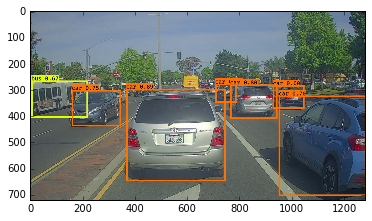Expected Output:

 Found 7 boxes for test.jpg car 0.60 (925, 285) (1045, 374) car 0.66 (706, 279) (786, 350) bus 0.67 (5, 266) (220, 407) car 0.70 (947, 324) (1280, 705) car 0.74 (159, 303) (346, 440) car 0.80 (761, 282) (942, 412) car 0.89 (367, 300) (745, 648)

The model you’ve just run is actually able to detect 80 different classes listed in “coco_classes.txt”. To test the model on your own images:
1. Click on “File” in the upper bar of this notebook, then click “Open” to go on your Coursera Hub.
2. Add your image to this Jupyter Notebook’s directory, in the “images” folder
3. Write your image’s name in the cell above code
4. Run the code and see the output of the algorithm!

If you were to run your session in a for loop over all your images. Here’s what you would get: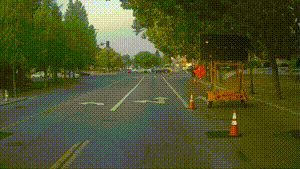Predictions of the YOLO model on pictures taken from a camera while driving around the Silicon Valley
Thanks drive.ai for providing this dataset!

What you should remember:
- YOLO is a state-of-the-art object detection model that is fast and accurate
- It runs an input image through a CNN which outputs a 19x19x5x85 dimensional volume.
- The encoding can be seen as a grid where each of the 19x19 cells contains information about 5 boxes.
- You filter through all the boxes using non-max suppression. Specifically:
- Score thresholding on the probability of detecting a class to keep only accurate (high probability) boxes
- Intersection over Union (IoU) thresholding to eliminate overlapping boxes
- Because training a YOLO model from randomly initialized weights is non-trivial and requires a large dataset as well as lot of computation, we used previously trained model parameters in this exercise. If you wish, you can also try fine-tuning the YOLO model with your own dataset, though this would be a fairly non-trivial exercise.

References: The ideas presented in this notebook came primarily from the two YOLO papers. The implementation here also took significant inspiration and used many components from Allan Zelener’s github repository. The pretrained weights used in this exercise came from the official YOLO website.
- Joseph Redmon, Santosh Divvala, Ross Girshick, Ali Farhadi - You Only Look Once: Unified, Real-Time Object Detection (2015)
- Joseph Redmon, Ali Farhadi - YOLO9000: Better, Faster, Stronger (2016)
- Allan Zelener - YAD2K: Yet Another Darknet 2 Keras
- The official YOLO website (https://pjreddie.com/darknet/yolo/)

Car detection dataset:The Drive.ai Sample Dataset (provided by drive.ai) is licensed under a Creative Commons Attribution 4.0 International License. We are especially grateful to Brody Huval, Chih Hu and Rahul Patel for collecting and providing this dataset.